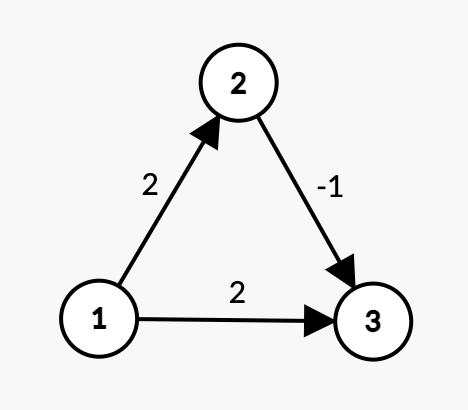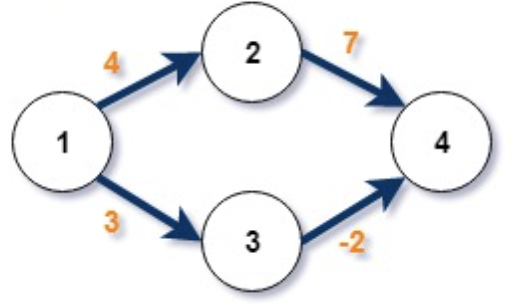2

# Bellman Ford

Difficulty: MEDIUMContributed By
Hardik Aggarwal
Avg. time to solve
30 min
Success Rate
70%

Problem Statement
Suggest Edit

#### Your task is to find the length of the shortest path between the ‘src’ and ‘dest’ vertex given to you in the graph. The graph may contain negatively weighted edges.

##### Example :``````3 3 1 3
1 2 2
1 3 2
2 3 -1
In the above graph, the length of the shortest path between vertex 1 and vertex 3 is 1->2->3 with a cost of 2 - 1 = 1.
``````

#### Note :

``````It's guaranteed that the graph doesn't contain self-loops and multiple edges. Also the graph does not contain negative weight cycles.
``````

#### Input Format :

``````The first line of input contains an integer ‘T’ denoting the number of test cases. Then each test case follows.

The first line of each test case contains four single space-separated integers ‘N’,  ‘M’ , ‘src’ and ‘dest’ denoting the number of vertices, the number of edges in the directed graph the source vertex and the destination vertex respectively.

The next ‘M’ lines each contain three single space-separated integers ‘u’, ‘v’, and ‘w’, denoting an edge from vertex ‘u’ to vertex ‘v’, having weight ‘w’.
``````

#### Output Format :

``````For each test case, return an integer denoting the length of the shortest path from ‘src’ to ‘dest’. If no path is possible return 10^9.
``````
##### Note :
``````You do not need to print anything, it has already been taken care of. Just implement the given function.
``````

#### Constraints :

``````1 <= T <= 10
1 <= N <= 50
1 <= M <= 300
1 <= src, dest <= N
1 <= u,v <= N
-10^5 <= w <= 10^5

Time Limit: 1 sec
``````
##### Sample Input 1 :
``````1
4 4 1 4
1 2 4
1 3 3
2 4 7
3 4 -2
``````
##### Sample Output 1 :
``````1
``````
##### Explanation For Sample Output 1 :``````The optimal path from source vertex 1 to destination vertex 4 is 1->3->4 with a cost of 3 - 2 = 1.
``````
##### Sample Input 2 :
``````1
2 1 1 2
2 1 3
``````
##### Sample Output 2 :
``````1000000000
``````Console